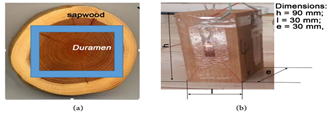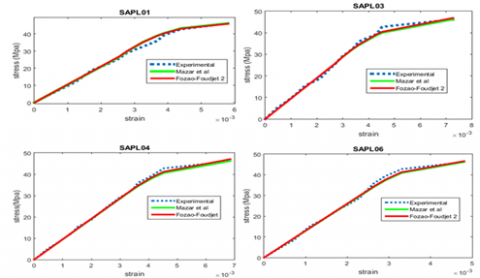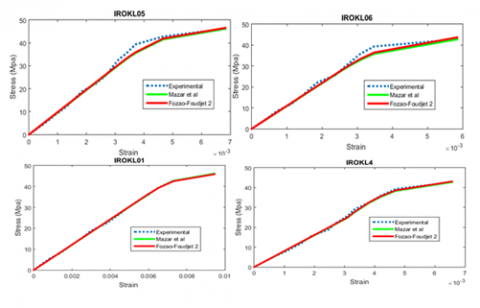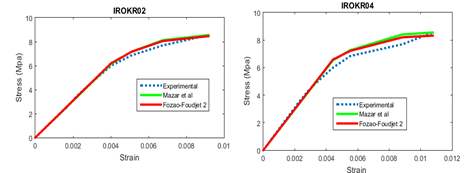# Modeling of the Stress-Strain Relationship of Wood Material Beyond Its Elasticity Limit under Cyclic Compressive Loading: Comparative Study of Two Models

Modeling of the Stress-Strain Relationship of Wood Material Beyond Its Elasticity Limit under Cyclic Compressive Loading: Comparative Study of Two Models

Thierry FotheUlrich Gael Azeufack Bienvenu Kenmeugne Pierre Kisito Talla Médard Fogue

Reasearch Unit of Mechanics and Physical Systems Modeling (UR2MPS), Department of Physics, University of Dschang, Dschang P.O.Box 67, Cameroon

Research Unit of Engineering of Industrial Systems and Environment (URISIE), UIT-FV Bandjoun, University of Dschang, Dschang P.O.Box 134, Cameroon

Laboratoire d’Engineering Civil et Mecanique (LECM), Ecole Nationale Superieure Polytechnique de Yaoundé, Yaoundé P.O.Box 8390, Cameroon

Corresponding Author Email:
thierryfothe@gmail.com
Page:
64-70
|
DOI:
https://doi.org/10.18280/mmep.080108
2 November 2020
|
Accepted:
26 January 2021
|
Published:
28 February 2021
| Citation

OPEN ACCESS

Abstract:

In this work, we study the relative capacities of two mathematical models for the description of the stress-strain relationship of wood material subjected to damaging cyclic compressive stresses. The models studied are the Mazars-Pijeaudier Cabot model and the Fozao-Foudjet model (Fozao-Foudjet 2). The envelope curves obtained experimentally during the cyclic compression tests were approximated using the two mathematical models and the coefficient determination R2 was calculated for each of the models. It appears from the observations that the two models present a good fit to the experimental results. In addition, the Fozao-Foudjet 2 model used with the parameters and chosen such as 0.00≤λ0≤0.015 and 0.99≤λ1≤1.05, shows a better fit to the experimental results compared to the Mazar-Pijeaudier Cabot model.

Keywords:

wood, cyclic compression, envelope curves, damage, mathematical models

1. Introduction

Wood is a biological material with impressive mechanical properties whose availability and ease of processing have made it the most used material for centuries. It operates at several levels in civil engineering, shipbuilding and many other fields. Its use has seen renewed interest in recent years due to the need to promote sustainable development while respecting the environment. The use of a material in the field of mechanical construction, whether in civil engineering, shipbuilding or simply carpentry, requires a prior knowledge of the mechanical characteristics of said material. These characteristics include the physicochemical properties of the material, the mechanical properties (Young's modulus, failure load, etc.) and the likely behaviors that these materials may exhibit when subjected to certain types of common stresses. The mastery of said characteristics not only guarantees better safety conditions, but also makes optimum use of the material. The study of the material in order to guarantee better safety conditions goes through the description of the various deterioration and rupture mechanisms of this material.

A material will be well known and therefore well valued if its different plasticity and damage mechanisms are well described. This problem is the subject of several studies carried out all over the world on the material wood [1-10]. The purpose of this study is to study the stress-strain relationship of some tropical species when they are stressed beyond their elasticity limits by cyclic compressive stresses.

It is shown that beyond the yield strength, a material exhibits nonlinear behavior. This nonlinearity is attributed mainly to the phenomena of plasticity and damage. These two phenomena are sufficiently well described by damage theory [11, 12].

Damage is the process of gradual deterioration leading to failure of the material , it begins with birth followed by the growth and coalescence of micro defects. The coalescence of the micro defects is the final step in the damage process and results in the appearance within the material of macroscopic cracks, thus marking the rupture of the material . The first models for describing damage appeared in the 1960s when Kachanov  defined a mathematical variable for describing damage. Much work has therefore emerged on the modeling of damage to materials such as metals and concrete [11-16]. The modeling of the damage is generally done within the framework of the mechanics of the continuous damage developed by the authors such as Lemaitre and Chaboche  whose essential points are: (1) the choice of an admissible thermodynamic potential for the establishment of the constitutive laws of the observable variables (strain, and temperature) and (2) the appropriate choice of a potential of dissipation for the evolution of the internal variables (plasticity, damage). The difference between the damage models thus proposed can therefore mainly reside in the choice of the dissipation potentials and therefore in the choice of the form of evolution of the damage variable. This choice is, however, guided by experimental observations and must be made in ways that best reproduce these experimental observations . There are thus many damage models in the literature which all differ from each other in the form of the law of evolution chosen for the damage [12, 14, 17]. However, the choice of a law of evolution being conditioned by experimental observation, it depends not only on the type of material studied, but also and above all on the type of stresses. The damage models are therefore developed according to the types of stress [1, 14].

One of the stress regimes responsible for material damage is the cyclic stress regime. These cyclic stresses can be low in amplitude in which case the damage is due to fatigue of the material after a high number of cycles; just as they can be of increasing amplitude and relatively high in which case damage occurs even after a low number of cycles . This second type of solicitation is the subject of this study.

The modeling of the damage under cyclic load with low number of cycles was the subject of numerous works on materials such as steels and concretes. [11-17] Despite the large number of models existing for the description of the behavior cyclic of materials such as concrete and steels, there is to our knowledge very little model describing the behavior of wood material under cyclic stress at low number of cycles [18-21]. The objective of this study is to find, among two existing damage models for modeling the behavior of materials other than wood, the one capable of best describing the cyclic behavior at low number of cycles of wood.

Many damage models have been proposed within the framework of the modeling of cyclic behaviors, a large part of these models, based on the theories of plasticity and damage; although efficient remain difficult to apply to materials, this mainly due to the large number of parameters required for their use. A model widely used for its relative simplicity is that proposed by Mazars and pijaudier-cabot . It was developed by the authors for the modeling of concrete damage. This model was subsequently adopted in the literature by many authors such as: Faria; Sima; Brecolleti [23-25], among many others for modeling concrete damage under cyclic stress. Recently it has been adopted in the framework of wood damage modeling by authors such as Wang . The initial model as proposed by the authors is sometimes modified in order to bring improvements in the description of the damage Sima [23-28], but these modifications all the time bring an additional number of parameters and make the model more complex and therefore more difficult to use. One of the recent modifications made to the model is that carried out by Fozao-Foudjet  for the description of behavior of bamboo under cyclic stress. The model named Fosao-Foudjet 2 thus proposed by the authors has been shown to be more suitable for modeling the stress-deformation curves of bamboo under cyclic stress. The adoption of one or the other of these models within the framework of the modeling of the cyclic behavior of wood should be done by taking into account the capacity of the model to accurately describe the behavior studied, in this case the stress-strain relation. In this study, we are therefore interested in a comparison of the two damage models in their capacity to describe the stress-strain relationship through the envelope curves of the wood material subjected to cyclical stress. In our work, we plot the envelope curves proposed by the Mazar-Pijodiercabot and Fozao-Foudjet models, which we compare to the envelope curves obtained experimentally.

It is shown that the envelope curve of the material subjected to cyclic compression can be approximated by a stress-strain curve of monotonic compression. This monotonic compression curve includes: a linear part (zone with elastic behavior) and a nonlinear part describing the nonlinear behavior. The envelope curve is therefore generally defined by a set of parameters obtained experimentally including (1) the initial modulus of elasticity, (2) the strain at the elastic limit, (3) the coordinates at the top of the stress-strain curve, as can be seen in Figure 1.

## 1.pngFigure 1. Typical monotonic uniaxial stress-strain curve [23, 26]

2. Presentation of the Study Models and Experimental Methods

2.1 Presentation of models

The models object of our study are those of Mazar-pijaudier –Cabot  and Fozao-Foudjet . The Mazar-Pijaudiercabot model was proposed for the description of the behavior of concrete under cyclic stress.The Fozao-Foudjet 2 model is obtained from the Mazar-pijaudiercabot model by the introduction of two parameters λ0 and λ1 with 1.00≤λ0≤1.38 and 0.0≤λ1≤0.13. For the description of the stress-strain relationship of bamboo. The mathematical expressions of the mazars-pijaudiercabot and Fozao-Foudjet 2 models are presented in Table 1. The original parameters were rewritten in terms of parameters obtained in the monotonic 1 D compression tests [23, 24].

Table 1. Mathematical expressions from Mazar-Pijeaudier-Cabot and from Fozao-Foudjet2 models

 Equations of Mazar - Pijaudier cabot’s models Equation of Fozao-Foudjet’s models 1 $\sigma =(1-D){{E}_{0}}\varepsilon$ $\sigma ={{\left( \frac{{{\varepsilon }_{c}}}{{{\varepsilon }_{0}}} \right)}^{{{\lambda }_{1}}}}(1-D){{E}_{0}}\varepsilon$ with 0.0≤λ1≤0.13 2 $D=1-\frac{{{\varepsilon }_{0}}}{\varepsilon }(1-B)-B\exp (\frac{{{\varepsilon }_{0}}-\varepsilon }{{{\varepsilon }_{c}}})$ $D=1-\frac{{{\varepsilon }_{0}}}{\varepsilon }(1-B)-B\exp (\frac{{{\varepsilon }_{0}}-\varepsilon }{{{\varepsilon }_{c}}})$ 3 $B=\frac{\left[ {{\sigma }_{c\max }} \right.-\left. {{E}_{0}}{{\varepsilon }_{0}} \right]}{{{E}_{0}}\left[ {{\varepsilon }_{c}}\exp (\frac{{{\varepsilon }_{0}}-{{\varepsilon }_{c}}}{{{\varepsilon }_{c}}})-{{\varepsilon }_{0}} \right]}$ $B=\frac{\left[ {{\sigma }_{c\max }} \right.-\left. {{E}_{0}}{{\varepsilon }_{0}} \right]}{{{E}_{0}}{{\varepsilon }_{0}}\left[ {{\left( {{k}_{0}} \right)}^{{{\lambda }_{0}}}}\exp (\frac{{{\varepsilon }_{0}}-{{\varepsilon }_{c}}}{{{\varepsilon }_{c}}})-1 \right]}$ With ${{k}_{0}}=\frac{{{\varepsilon }_{c}}}{{{\varepsilon }_{0}}}$ and 1.00≤λ0≤1.38

In these formulas,

σ is the applied stress;

•${{\sigma }_{c\max }}$ is the maximum applied stress;

•${{E}_{0}}$ is the modulus of elasticity;

D is the Damage of the material;

•$\varepsilon$ is the total strain;

•${{\varepsilon }_{0}}$ is the elastic strain;

•${{\varepsilon }_{c}}$ is the strain at the maximum applied stress;

λ0 and λ1 are parameters of Fozao-Foudjet 2 model.

## 2.pngFigure 2. Zone of extraction of specimen (a) and dimensions of specimen (b)

Wood is a biological, anisotropic, heterogeneous and hygroscopic material. Each species of wood has its own physical properties. In addition, theses physicals and mechanicals properties are all dependent on its moisture content. The knowledge of a mechanical behavior of wood begins with the knowledge of its moisture content and its intrinsic mechanical caracteistics (modulus of elasticity and the failure load ect). The species studied are sapelli and iroko. The 30mmx30mmx90mm compression specimens were taken from the heartwood of each species according to standard NFB 51-003, Figure 2(a) shows the zone of extraction, while Figure 2(b) shows the dimensions of the specimen. The determination of the moisture content was made following the standard NFB 51-004. The moisture content is given by the relation:

$H=\frac{{{m}_{H}}-{{m}_{0}}}{{{m}_{0}}}x100$     (1)

where:

·H is the moisture content of the specimen;

·m0 is the mass of wood in an anhydrous state;

·mH is the mass of wood with moisture H.

The average humidity rate for our essences obtained during the tests was 19% for the sapelli and 15.82% for the iroko.

3. Results and Discussions

The envelope curves of the cyclic compression tests obtained by Fothe et al.  were plotted and approximated by the models of Mazar-Pijaudiercabot and Fosao-Foudjet. It is observed that both models give a good approximation of the stress-strain curve for stress levels below the yield stress where the material exhibits linear behavior. For the zone with nonlinear behavior, we observe divergences between the approximations of the two models. These divergences depend on the parameters λ0 and λ1 chosen for the Fozao-Foudjet 2 model; For certain values of these parameters, 1 and 0 respectively, one obtains a superposition of the Fozao-Foudjet curves on the Mazar-pijaudiercabot curves. This is easily explained by the fact that the Fozao-Foudjet model derives from the Mazars model by the introduction of the parametersλ0 and λ1. For other values of these parameters, the divergence between these two models is such that the curves of the Fozao-Foudjet model are closer to the experimental curves than that of Mazar-pijaudiercabot; thus making the Fozao-Foudjet model more apt to describe the stress-strain relation than the Mazar-pijaudiercabot model. The following Figures 3 a-d represent the experimental envelope curves and those proposed by the two models for certain values of λ0 and λ1 defined such as 1.00≤λ0≤1.38 and 0.0≤λ1≤0.13. For specimens in different directions.

## 31.png(a) Measured and calculated data compared Sapelli in longitudinal Direction

## 32.png(b) Measured and calculated data compared Iroko in longitudinal Direction

## 33.png(c) Measured and calculated data compared Sapelli in Radial Direction

## 34.png(d) Measured and calculated data compared Iroko in Radial Direction

Figure 3. Measured and calculated data compared

The following Tables 2-5 present the values of the parameters used in the plotting of the curves of the preceding figures. The coefficient of determination R2  for the two models was calculated for each sample by the relation:

${{R}^{2}}=1-\frac{\sum\limits_{1}^{n}{(\sigma _{\exp }^{i}-\sigma _{cal}^{i})}}{\sum\limits_{1}^{n}{\left( \sigma _{\exp }^{i}-{{{\bar{\sigma }}}_{\exp }} \right)}}$     (2)

where:

•$\sigma _{\exp }^{i}$ is the experimental value of the stress;

• $\sigma _{cal}^{i}$is the value of the stress calculated by the model;

• ${{\bar{\sigma }}_{\exp }}$is the mean value of the experimental stress

The results are given in the following Tables 2-5.

It comes from the preceding Tables 2-5 that the values of the parameters and for each of the wood species and in each of the two directions are defined such that: 0.00≤λ0≤0.015 and 0.99≤λ1≤1.05. These values are lower than those proposed by Fozao-Foudjet in their study on bamboo. This difference can be explained by the fact that although they are both organic materials, wood and bamboo do not have the same cellular structure. The values of the coefficients of determination for each model and for all the test pieces in longitudinal directions of sapelli and iroko are such that R2≥98.86%. For the radial directions, this coefficient of determination for the great majority of specimens is such that: R2≥95.76%. These observations allow to conclude that the models of Mazar-PijaudierCabot and Fozao-Foudjet give good descriptions of the stress relation of formation of the wood material subjected to cyclic compressions.

However, for the entire test specimen, the coefficient of determination of the Fozao-Foudjet model is higher than that of theMazar-Pijaudiercabot model. This shows quantitatively that for the parameters and chosen such as 0.00≤λ0≤0.015 and 0.99≤λ1≤1.05. The Fozao-Foudjet 2 model gives a better description of the stress-strain relationship than the Mazar-Pijaudiercabot mode.

Table 2. Parameters used in the models for Sapelli in the longitudinal direction

 Mazar’s Equation Fozao-Foudjet 2 specimen E0(Mpa) σcmax (Mpa) $\varepsilon_{c}$(x10-3) $\varepsilon_{0}$(x10-3) $\lambda_{0}$ $\lambda_{1}$ $B$ R2(%) $\lambda_{0}$ $\lambda_{1}$ $B$ R2(%) SAPL01 10482.38 46.18 5.8 2.5 1.00 0.00 2.43 99.24 1.02 0.010 2.35 99.37 SAPL02 9392.17 46.18 7.00 2.70 1.00 0.00 2.03 99.86 1.01 0.010 1.96 99.86 SAPL03 9506.12 46.18 7.3 2.73 1.00 0.00 1.83 99.46 0.99 0.0001 1.89 99.58 SAPL04 9506.12 46.18 6.9 3.4 1.00 0.00 1.95 99.60 0.99 0.01 2.03 99.73 SAPL05 13584.54 46.18 4.9 2.1 1.00 0.00 1.90 99.71 0.99 0.010 1.90 99.74 SAPL06 12919.52 46.18 4.8 2.6 1.00 0.00 2.21 99.49 0.99 0.015 2.21 99.61

Table 3. Parameters used in the models for Iroko in the longitudinal direction

 Mazar’s Equation Fozao-Foudjet 2 specimen ${{E}_{0}}$(Mpa) ${{\sigma }_{c\max }}$(Mpa) ${{\varepsilon }_{c}}$(x10-3) ${{\varepsilon }_{0}}$(x10-3) ${{\lambda }_{0}}$ ${{\lambda }_{1}}$ $B$ R2(%) ${{\lambda }_{0}}$ ${{\lambda }_{1}}$ $B$ R2(%) IROKL01 6042.88 46.18 9.5 6.00 1.00 0.00 2.96 99.94 1.01 0.01 2.78 99.94 IROKL02 8068.25 42.76 6.4 4.4 1.00 0.00 3.17 99.79 1.00 0.01 3.17 99.77 IROKL03 9784.42 42.76 5.2 3.7 1.00 0.00 3.47 99.68 1.00 0.01 3.47 99.71 IROKL04 8002.97 42.76 6.6 3.1 1.00 0.00 2.89 99.69 1.00 0.01 2.89 99.75 IROKL05 9725.26 46.18 6.8 3.1 1.00 0.00 1.92 98.88 1.00 0.01 1.92 99.06 IROKL06 10862.97 42.76 5.8 2.6 1.00 0.00 1.76 98.86 0.99 0.01 1.83 98.99

Table 4. Parameters used in the models for Sapelli in the radial direction

 Mazar’s Equation Fozao-Foudjet 2 specimen ${{E}_{0}}$ (Mpa) ${{\sigma }_{c\max }}$(Mpa) ${{\varepsilon }_{c}}$(x10-3) ${{\varepsilon }_{0}}$(x10-3) ${{\lambda }_{0}}$ ${{\lambda }_{1}}$ $B$ R2(%) ${{\lambda }_{0}}$ ${{\lambda }_{1}}$ $B$ R2(%) SAPR01 1424.60 13.68 15.9 4.8 1.00 0.00 1.53 94.99 1.02 0.01 1.44 95.76 SAPR02 1485.28 13.68 10.00 4.5 1.00 0.00 3.73 90.72 0.99 0.01 3.78 91.77 SAPR03 1592.15 13.68 15.87 4.4 1.00 0.00 1.26 97.10 1.02 0.01 1.26 97.51 SAPR04 1339.33 11.11 12.00 4.9 1.00 0.00 1.95 99.31 1.02 0.01 1.82 99.59 SAPR05 1321.76 11.11 11.3 5.3 1.00 0.00 2.32 97.21 1.02 0.01 2.15 97.80

Table 5. Parameters used in the models for Iroko in the radial direction

 Mazars Equation Fozao-Foudjet 2 specimen ${{E}_{0}}$(Mpa) ${{\sigma }_{c\max }}$(Mpa) ${{\varepsilon }_{c}}$(x10-3) ${{\varepsilon }_{0}}$(x10-3) ${{\lambda }_{0}}$ ${{\lambda }_{1}}$ $B$ R2(%) ${{\lambda }_{0}}$ ${{\lambda }_{1}}$ $B$ R2(%) IROK R01 1227.35 8.55 13.6 4.9 1.00 0.00 0.91 99.28 1.01 0.00 0.86 99.29 IROK R02 1531.48 8.55 9.20 4.00 1.00 0.00 1.29 99.46 1.02 0.01 1.20 99.51 IROK R03 739.67 8.55 19.2 8.4 1.00 0.00 1.24 99.38 1.02 0.01 1.16 99.36 IROK R04 1474.80 8.55 10.8 4.4 1.00 0.00 0.88 98.19 1.05 0.01 0.75 98.49 IROK R05 1058.14 8.55 16.4 5.8 1.00 0.00 0.81 99.69 1.00 0.00 0.76 99.73
4. Conclusions

This work investigated the relative capacities of two damage models to describe the stress-strain relationship of wood materials subjected to cyclic compressive stresses. The models object of this study are the models of Mazar-Pijaudier Cabot and the model of Fozao-Foudjet 2. The experimental envelopes curves obtained from cyclic compression tests on the longitudinal and radial directions of the sapelli and the iroko were drawn and compared to the envelope curves proposed by each of the two models. The calculation of the coefficient of determination R2for each of the models was also carried out.

The results show a good agreement between the experimental curves and the two mathematical models. For the Fozao-Foudjet 2 models, this good agreement is obtained from the parameters λ0 and λ1 chosen as 0.00≤λ0≤0.015 and 0.99≤λ1≤1.05. The parameters of the model of Fozao-Foudjet 2, retained for the modeling of the cyclic compression of wood are thus defined such as 0.00≤λ0≤0.015 and 0.99≤λ1≤1.05.

Moreover, for the parameters and chosen such as 0.00≤λ0≤0.015 and 0.99≤λ1≤1.05. The coefficient of determination for the Fozao-Foudjet 2 model is greater than that of the Mazar-Pijaudiercabot model for all of the two directions. This makes the Fozao-Foudjet 2 model a better candidate for describing the behavior of wood material subjected to cyclic compressions.

References

 Guitar, D. (1987). Mécanique du Matériau Bois et Composite, Collection nabla.

 Kollmann, F.F.P., Cote, W.A. (1968). Principles of Wood Science and Technology. Volume 1, Solid Wood. Springer-Verlag, New York.

 Van Der Put. (1989). Deformation and Damage Processes in Wood. Delft University Press. http://resolver.tudelft.nl/uuid:ac2ea262-be4d-48c2-9f10-f35bd7c14a05

 Thibaut, B., Gril, J., Fournier, M. (2001). Mechanics of wood and trees: Some new highlights for an old story. C. R. Acad. Sci. Paris, t. 329, Série II b, 701-716.

 Aira, J.R., Íñiguez-González, G. (2014). Determination of the elastic constants of scots pine (pinus sylvestris l.) wood by means of compression tests. Biosysteme Engineering, 1261: 2-22. https://doi.org/10.1016/j.biosystemseng.2014.07.008

 Nziengui, C.F.P., Ikogou, S., Pitti, R.M. (2017). Impact of cyclic compressive loading and moisture content on the mechanical behavior of Aucoumea Klaineana Pierre. Wood Material Science & Engineering, 13(4): 190-196. https://doi.org/10.1080/17480272.2017.1307281

 Edzang, A.C.E., Nziengui, C.F.P., Ango, S.E., Ikogou, S., Pitti, R.M. (2020). Comparative studies of three tropical wood species under compressive cyclic loading and moisture content changes, Wood Material Science & Engineering. https://doi.org/10.1080/17480272.2020.1712739

 Houanou, A.K., Tchéhouali, A.D., Foudjet, A.E. (2014). Effect of the loading duration on the linear viscoelastic parameters of tropical wood: Case of Tectona grandis L.f (Teak) and Diospyros mespiliformis (Ebony) of Benin Republic. Springer Plus 3: 74. https://doi.org/10.1186/2193-1801-3-74

 Foadieng, E., Fogue, M., Talla, P.K., Ntabe, E. (2017). Effets de la Variation du Taux d’Humidité sur les Propriétés Mécaniques de Mélicia exelcia (iroko) 6ieme journées du grd 3544 « sciences du bois » Nantes pp 56-57. https://hal-enpc.archives-ouvertes.fr/hal-01713401

 Azeufack, U.G., Kenmeugne, B., Foadieng, E. Fouotsa, M., Talla, P.K., Fogue, M. (2019). Mechanical characterization and measurement of damage of Pericopsis Elata (Assamela). World Journal of Engineering and Technology, 7(2): 256-269. https://doi.org/10.4236/wjet.2019.72017

 Lemaitre, J. (1996). A Course on Damage Mechanics. Springer-Verlag. https://doi.org/10.1007/978-3-642-18255-6

 Lemaitre, J., Desmorat, R. (2005). Engineering Damage Mechanics. Springer. https://doi.org/10.1007/b138882

 Lemaitre, J., Chaboche J.L., Benalal, A., Desmorat, R. (2009). Mécanique des Matériaux Solides, Dunod.

 Murakami, S. (2012). Continium Damage Mechanics. Springer-Verlag. https://doi.org/10.1007/978-94-007-2666-6

 Kachanov, L.M. (1958). Time of rupture process under creep conditions. Otdelenie Tekhniecheskichnauk, 8: 26-31. https://ci.nii.ac.jp/naid/10030415483/en/.

 Lemaitre, J., Dufailly, J. (1987). Damage measurements. Engineering Fracture Mechanics, 28(5-6): 643-647. https://doi.org/10.1016/0013-7944(87)90059-2Get

 Meng, Q., Wang, Z. (2016). Creep damage models and applications for crack growth analysis in pipes: A review. Engineering Fracture Mechanics. http://dx.doi.org/10.1016/j.engfracmech.2015.09.055

 Wang, M.Q., Song, X.B., Gu, X.L. (2018). Three-dimensional combined elasto-plastic and damage model for nonlinear analysis of wood. American Society of Civil Engineers, 144(8). https://doi.org/10.1061/(ASCE)ST.1943-541X.0002098

 Sandhaas, C. (2011). 3D material model for wood, based on continuum damage mechanics. Stevinrapport 6-11-4, Stevin II Laboratory. Delft University of Technology, The Netherlands.

 Khelifa, M., Khennane, A., El Ganaoui, M., Celzard, A. (2016). Numerical damage prediction in dowel connections of wooden structures. Mater Struct., 49: 1829-1840. https://doi.org/10.1617/s11527-015-0615-5

 Khennane, A., Khelifa, M., Bleron, L., Viguier, J. (2014). Numerical modelling of ductile damage evolution in tensile and bending tests of timber structures. Mech Mater., 68: 228-236. https://doi.org/10.1016/j.mechmat.2013.09.004

 Mazars, J., Pijaudier-Cabot, G. (1989). Continuum damage theory. Application to concrete. J Engng Mech ASCE, 115(2): 345-365. https://doi.org/10.1061/(ASCE)0733-9399(1989)115:2(345)

 Sima, J.F., Roca, P., Molins, C. (2007). Cyclic constitutive model for concrete. Engineering Structures, 30(3) 695-706. https://doi.org/10.1016/j.engstruct.2007.05.005

 Faria, R., Oliver, J., Cervera, M. (1998). A strain-based plastic viscous-damage model for massive concrete structures. Int J Solids Struct., 35(14): 1533-58. https://doi.org/10.1016/S0020-7683(97)00119-4

 Breccolotti, M., Bonfigli, M.F., D’Alessandro, A., Materazzi, A.L. (2015). Constitutive modeling of plain concrete subjected to cyclic uniaxial compressive loading. Construction and Building Materials, 94: 172-180. https://doi.org/10.1016/j.conbuildmat.2015.06.067

 Fozao, D.S., Foudjet, A.E. (2020). Stress-strain behaviour of bamboo beyond its elastic limit under uniaxial compressive load, using the principles of plasticity and damage mechanics. Revue Scientifque et Technique Forêt et Environnement du Bassin du Congo, 14: 12-20. http://doi.org/10.5281/zenodo.3737878

 Babu, Y.S., Reddy, V.S., Raju, S.P.V., Rao, M.V.S. (2020). A review of mathematical models for prediction of Stress-strain and moment –curvature behaviour in concrete. E3S Web of Conferences, 184: 01111. https://doi.org/10.1051/e3sconf/202018401111

 Kaklis, K., Agioutantis, Z., Mavrigiannakis, S., Maravelaki-Kalaitzaki, P. (2019). A simplified damage evolution relationship and deformation characteristics of a pozzolanic lime mortar when subjected to unloading-reloading cycles in the pre-peak region. Frattura ed Integrità Strutturale, 50: 395-406. https://doi.org/10.3221/IGF-ESIS.50.33

 Fothe, T., Azeufack, U.G., Kenmeugne, B., Talla, P.K., Fogue, M. (2019). Damage evaluation of two equatorial hardwoods under uniaxial compression: Case of Entandrophragma cylindricum (Sapelli) and Chlorophora exelcia (Iroko). World Journal of Engineering and Technology, 7: 549-558. https://doi.org/10.4236/wjet.2019.74039